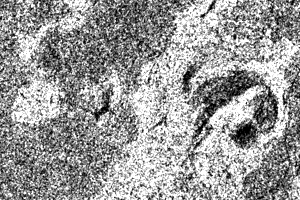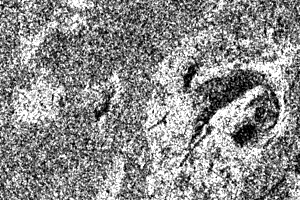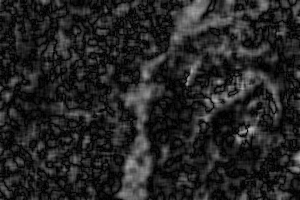# RatioChDet.cxx¶

This example illustrates the class otb::MeanRatioImageFilter for detecting changes between pairs of images. This filter computes the mean intensity in the neighborhood of each pixel of the pair of images to be compared and uses the ratio of means as a change indicator. This change indicator is then normalized between 0 and 1 by using the classicalwhereandare the local means.Radarsat fine mode acquisitions before (left) and after (middle) a lava flow resulting from a volcanic eruption. On the right is the result of the change detection by ratio of local means.

Example usage:

./RatioChDet Input/GomaAvant.png Input/GomaApres.png Output/RatioChDet.tif 3


Example source code (RatioChDet.cxx):

#include "otbMeanRatioImageFilter.h"
#include "otbImageFileWriter.h"
#include "otbImage.h"
#include "itkShiftScaleImageFilter.h"
#include "otbCommandProgressUpdate.h"

int main(int argc, char* argv[])
{

if (argc < 5)
{
std::cerr << "Usage: " << std::endl;
std::cerr << argv << " inputImageFile1 inputImageFile2  outputImageFile radius" << std::endl;
return -1;
}

// Define the dimension of the images
const unsigned int Dimension = 2;

// We start by declaring the types for the two input images, the
// change image and the image to be stored in a file for visualization.
using InternalPixelType = float;
using OutputPixelType   = unsigned char;
using InputImageType1   = otb::Image<InternalPixelType, Dimension>;
using InputImageType2   = otb::Image<InternalPixelType, Dimension>;
using ChangeImageType   = otb::Image<InternalPixelType, Dimension>;
using OutputImageType   = otb::Image<OutputPixelType, Dimension>;

// We can now declare the types for the readers. Since the images
// can be vey large, we will force the pipeline to use
// streaming. For this purpose, the file writer will be
// streamed. This is achieved by using the
// ImageFileWriter class.
using WriterType  = otb::ImageFileWriter<OutputImageType>;

// The change detector will give a normalized result between 0 and
// 1. In order to store the result in PNG format we will
// rescale the results of the change detection in order to use all
// the output pixel type range of values.

// The MeanRatioImageFilter is templated over
// the types of the two input images and the type of the generated change
// image.
using FilterType = otb::MeanRatioImageFilter<InputImageType1, InputImageType2, ChangeImageType>;

// The different elements of the pipeline can now be instantiated.
WriterType::Pointer   writer   = WriterType::New();
FilterType::Pointer   filter   = FilterType::New();

const char* inputFilename1 = argv;
const char* inputFilename2 = argv;
const char* outputFilename = argv;

// We set the parameters of the different elements of the pipeline.
writer->SetFileName(outputFilename);
float scale = itk::NumericTraits<OutputPixelType>::max();
rescaler->SetScale(scale);

// The only parameter for this change detector is the radius of
// the window used for computing the mean of the intensities.

// We build the pipeline by plugging all the elements together.